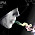Common mistakes students make when learning production theory - FreeEconHelp.com, Learning Economics... Solved!

## 8/8/12

This economics post goes over some of the most common mistakes that I have seen students make when turning in homework and completing exams for the production theory portion of introductory microeconomics.  Remember that this concept is what students will need to understand stand in order to perform well in all of the production theory chapters including perfect competition, monopolistic competition, oligopolies and monopolies.  Understanding the theory behind production and the associated relationships between all of the cost curves is very important and will lead to an easier time performing well in the later chapters.

First, when first learning about the relationships between all of the various costs -- marginal cost, variable cost, average variable cost, total cost, average total cost, fixed cost, and average fixed cost -- don't memorize the graphs without understanding the numbers that go on behind them.  It is very useful in the beginning to always have a graph or table with the associated numbers behind the graphs to refer to, especially if you have questions about the visual representation (the graph).  It is important to remember that each cost has an associated equation and relationship to the other costs, and it is this interaction that gives the graphs the curves that they do.

It will also help in the beginning to relate the labor graph (with product and marginal product) to the cost graph to truly understand the relationships between these two graphs. For example, it is the relationship between product and output that gives the marginal cost curve the shape it has which then drives the shape for most of the other cost curves present in the cost graph.

Second, there are a lot of new graphs and relationships introduced in this chapter and it can be easy to be overwhelmed by them.  It is important to go through each graph individually and construct them from scratch to understand what they are trying to show and how they all relate to each other.  I would recommend beginning with the labor productivity graph, graphing labor inputs and the associated product produced.  We know that at the maximum of AP (average product) the marginal cost will be at its minimum.  We also know that MC (marginal cost) will intersect AC (average cost) AVC (average variable cost) and ATC (average total cost) at their minimum points from below.  If you can remember these important points, you should always be able to double check your graphs to make sure you drew them correctly.

Third, keep the ideas of short run vs. long run clear.  The reasons are different for the U shaped SRATC (short run average total cost) curve and the LRATC (long run average total cost) curve.  The SRATC curve is U shaped because of decreasing marginal product while the LRATC curve is U shaped because of increasing, constant, and decreasing returns to scale.  Diseconomies of scale are not related to diminishing labor productivity because both labor and capital can change in the long run.  Diseconomies of scale occur due to size of the firm resulting in organizational chaos or other management style inefficiencies that occur in large operations.

Finally, it is important to understand the relationships between marginal and average variables.  Review the prior economics post on the relationship between marginal and average variables for a good review.

1.2.3.